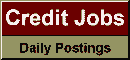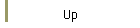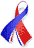DefaultRisk.com the web's biggest credit risk modeling resource.doi> search: A or B Export citation to:- HTML- Text (plain)- BibTeX- RIS- ReDIF

Default Correlations and the Effect of Estimation Errors on Risk Figures

by Christina R. Niethammer of the University of Konstanz, and
Ludger Overbeck of the University of Giessen

June 18, 2008

Abstract: In credit risk management, risk figures rely on single default probabilities and the dependence between the assets, usually modeled as correlation. The estimation of these parameters depends on the given data and with those certain implied risk figures. Parameter estimates change if data change, but how? Using the modeled distribution of the data we obtain asymptotic distributions for our parameter estimates describing the extent of these deviations. Taking these asymptotic distributions, we derive confidence intervals for our risk figures, namely probabilities of a percentage loss in a credit portfolio, the expected shortfall of Vasicek's loss distribution, the expected loss of a CDO-tranche etc...

Keywords: default correlation, risk management, Gaussian copula model, confidence intervals.

Previously titled: Asset Correlations and the Effect of Estimation Errors on Risk Figures

Books Referenced in this paper:  (what is this?)